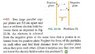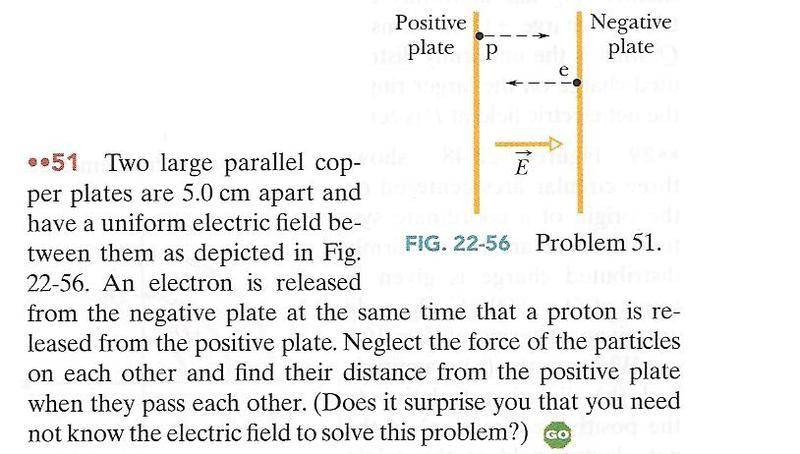# Uniform Electric Field

• jegues

## Homework Statement

See figure attached for problem statement.

## The Attempt at a Solution

I don't really know how to start this problem. What should I be thinking about in this problem?

We've never done a problem of this type so I'm having a hard time getting started.

Thanks again!

#### Attachments

•CH22-Q51.JPG
53.3 KB · Views: 495

hi jegues!find the acceleration of each particle (for that, you need to find the force) …

what do you get?## Homework Statement

See figure attached for problem statement.

## The Attempt at a Solution

I don't really know how to start this problem. What should I be thinking about in this problem?

We've never done a problem of this type so I'm having a hard time getting started.

Thanks again!The electric field, E, is uniform and points to the right.

What force, F, does E exert on a particle with charge, q ? ... on a particle with charge, -q ?

What is the acceleration, a, of a particle of mass, m, if a force, F, is exerted on it?

If the proton is a distance, x, from the positive plate at the time it passes the electron, how far is the electron from the negative plate at the same time?

What is the ratio of the mass of a proton to the mass of an electron?

hi jegues!find the acceleration of each particle (for that, you need to find the force) …

what do you get?How do we go about getting the force on each particle?

I know each plate is going to push their respective particle away but I don't see how I can calculate the exact force.

Can you give me another nudge?The electric field, E, is uniform and points to the right.

What force, F, does E exert on a particle with charge, q ? ... on a particle with charge, -q ?

$$\vec{F = \vec{E}q \text{, or for -q, } - \vec{E}q }$$
What is the acceleration, a, of a particle of mass, m, if a force, F, is exerted on it?

$$\vec{a} = \frac{\vec{E}q}{m}$$

If the proton is a distance, x, from the positive plate at the time it passes the electron, how far is the electron from the negative plate at the same time?

$$0.05 - x$$

What is the ratio of the mass of a proton to the mass of an electron?[/QUOTE]

$$\frac{m_{p}\ =\ 1.67262158(13)\ \times\ 10^{-27}\ kg}{m_{e}\ =\ 9.10938188(72)\ \times\ 10^{-31}\ kg}$$

Thanks for the help! I hope I answered all of the questions you posed correctly. I didn't know the vector E given in the diagram was actually correct I thought there would be another electric field due to the negative plate. (What am I confusing?)

I'll work at trying to solve my final answer and post my results.

EDIT: Okay so first the acceleration of the proton,

$$\vec{a_{p}} = \frac{\vec{E}q_{p}}{m_{p}}, \text{ where } q_{p} = 1.602176 \times 10^{-19} C, \quad m_{p} = 1.67262158(13)\ \times\ 10^{-27}\ kg$$

now electron,

$$\vec{a_{e}} = \frac{\vec{E}q_{e}}{m_{e}}, \text{ where } q_{e} = -1.602176 \times 10^{-19} C, \quad m_{e} = 9.10938188(72)\ \times\ 10^{-31}\ kg$$

I still can't quite see how to put things together to obtain my final equation where I can solve for the distance x.

You asked about the ratio of the mass of the electron and proton and I was able to come up with this formula by setting my 2 equations for accelerations equal to each other. (They posses the same E)

$$-\frac{\vec{a_{e}}}{\vec{a_{p}}} = \frac{m_{p}}{m_{e}}$$

I'm not sure if that's what we should be working with or not...

Last edited:
Updated.

You've now got two uniformly accelerated projectiles. You know their starting positions and their accelerations. Essentially, since their mutual interactions are being ignored, you want to know where they'd meet. So, two equations of motion, one intersection.

Things are simplified if you know the ratio of the accelerations -- replace two variables with one (scaled of course).

...

$$\frac{m_{p}\ =\ 1.67262158(13)\ \times\ 10^{-27}\ kg}{m_{e}\ =\ 9.10938188(72)\ \times\ 10^{-31}\ kg}$$
≈1836
...

$$-\frac{\vec{a_{e}}}{\vec{a_{p}}} = \frac{m_{p}}{m_{e}}$$

I'm not sure if that's what we should be working with or not...

To simplify things, drop to signs and say, if the proton travels distance, x, then the electron travels distance, 0.05 - x. Also,

$$a_{e} = \frac{m_{p}}{m_{e}}\,a_{p}\approx 1836\,a_{p}\,.$$

Therefore:

$$x={{1}\over{2}}\,a_{p}\,t^2\ \text{ and }\ 0.05-x={{1}\over{2}}\,1836\,a_{p}\,t^2 \,.$$

Divide one equation by the other to eliminate t, or else solve the first for t2, plug that into the second equation. Then solve for x.

≈1836

To simplify things, drop to signs and say, if the proton travels distance, x, then the electron travels distance, 0.05 - x. Also,

$$a_{e} = \frac{m_{p}}{m_{e}}\,a_{p}\approx 1836\,a_{p}\,.$$

Therefore:

$$x={{1}\over{2}}\,a_{p}\,t^2\ \text{ and }\ 0.05-x={{1}\over{2}}\,1836\,a_{p}\,t^2 \,.$$

Divide one equation by the other to eliminate t, or else solve the first for t2, plug that into the second equation. Then solve for x.

Thank you all for your help. I was finally able to obtain the correct answer. However, I still have 1 question.

Why can we simply drop the sign in the ratio's between acceleration and mass?

Thank you all for your help. I was finally able to obtain the correct answer. However, I still have 1 question.

Why can we simply drop the sign in the ratio's between acceleration and mass?

It's not really all that different to drop the signs for the electron's acceleration (and displacement).

When you drop the signs, you're just working with the distance that the electron travels, distance being a positive quantity.

If you keep the negative sign with the electron's acceleration, then you need to be careful of the sign of the electron's displacement. In this case, the displacement of the electron is x-0.05. So you would have had:

x-0.05=(1/2)(1836)(-ap)t2.

It's not really all that different to drop the signs for the electron's acceleration (and displacement).

When you drop the signs, you're just working with the distance that the electron travels, distance being a positive quantity.

If you keep the negative sign with the electron's acceleration, then you need to be careful of the sign of the electron's displacement. In this case, the displacement of the electron is x-0.05. So you would have had:

x-0.05=(1/2)(1836)(-ap)t2.

That makes perfect sense.

Thanks again!